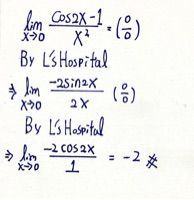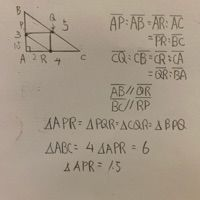# 109 年 - 109 台灣綜合大學系統學士班轉學生聯合招生試題：微積分A#96944

【非選題】
1.
1. (10 pts) Evaluate the following limits if they exist.

【題組】

(a)1F

【非選題】
2.【題組】

(b)1F【非選題】
3.2. (10 pts) A curve in ℝ2 is given parametrically by

x=t2+2t+3
y=t4-3t3
for all t＞0. Findat the point (6,-2).

1F

x = t²+2t+3 ->6    (t-1)(t+3)=0
y = t⁴-3t³     ->-2   (t-1)(t³-2t²-2t-2)=0
1 -3  0   0   2 ]
1 -2  -2  -2 ]  1
———————
1 -2 -2 -2 |_0
—————————————————

dy       dy/dt          4t³-9t² (t代入1)        -5
— = ———— = ——————— = —— = -5/4
dx       dx/dt           2t+2  (t代入1)           4
【非選題】
4.

3. (10 pts) Let x＞0 and △ABC be a triangle whose side lengths are. Choose a point P on, and a point Q on, and a point R onso thatLet f(x) be the area of △PQR . Find the critical point and the minimum of f(x).

1F

Critical Point x = 1
f(1) = 1.5【非選題】
5.

4. (10 pts) Find the radius of the convergence of the power series【非選題】
6.

5.(10 pts) Evaluate the improper integral【非選題】
7.

6. (10 pts) Let g :(0,∞)-be a twice differentiable function. Assume that
g(I)=I, g'(I)=3,g"(I)=-4.
Define a real valued function h on【非選題】
8.7.(10 pts) Let S be the surface defined by the equation
xcos(xy)+z2y4 -7xz =1
and P(0,1,1) be a point on S . Find an equation that defines the tangent plane to S at p and a parametric equation of the normal line to S at P.

【非選題】
9.

8. (10 pts) Evaluate the double integral【非選題】
10.

9. (10 pts) Let C be the curve indefined by the parametric equation
x(t)=cos(t),y(t)=sin(t),z(t)=t
for 0 ≤ t ≤ a. Suppose that the arc length of C is. Evaluate the line integalof the vector field F=【非選題】
11.

10. (10 pts) Find the flux of the vector field F ondefined bythrough the surface S =oriented with upward pointing normal vector field.